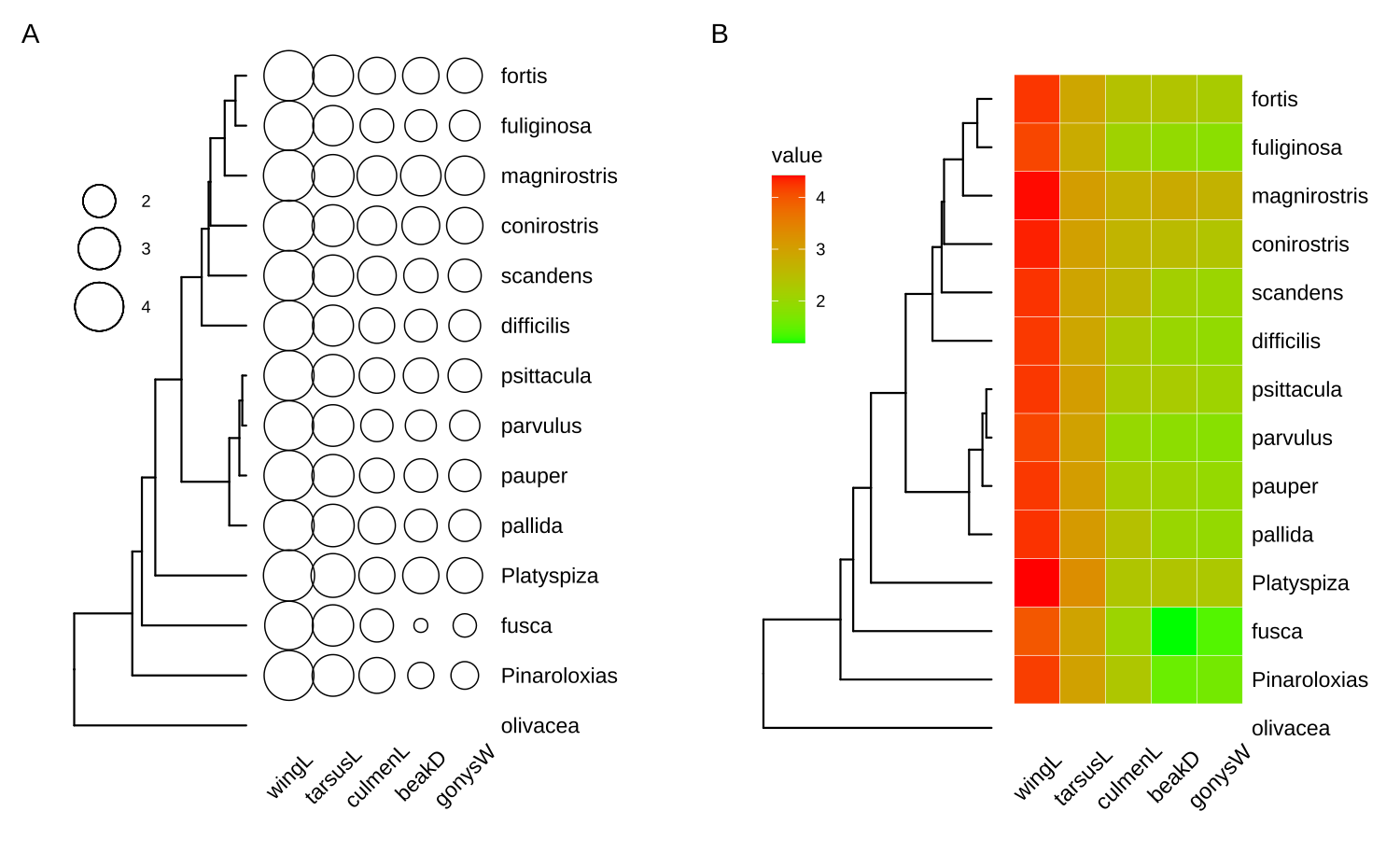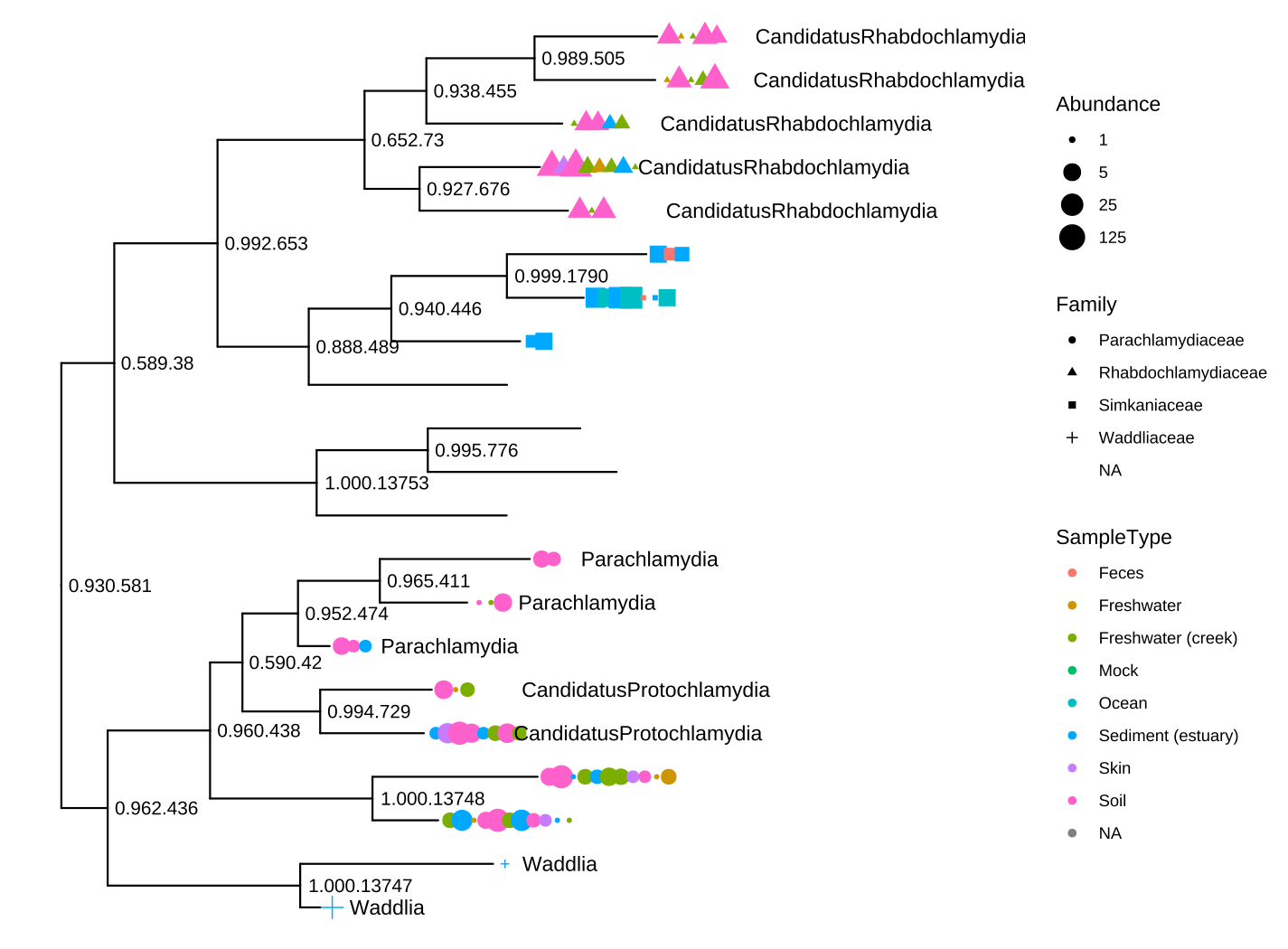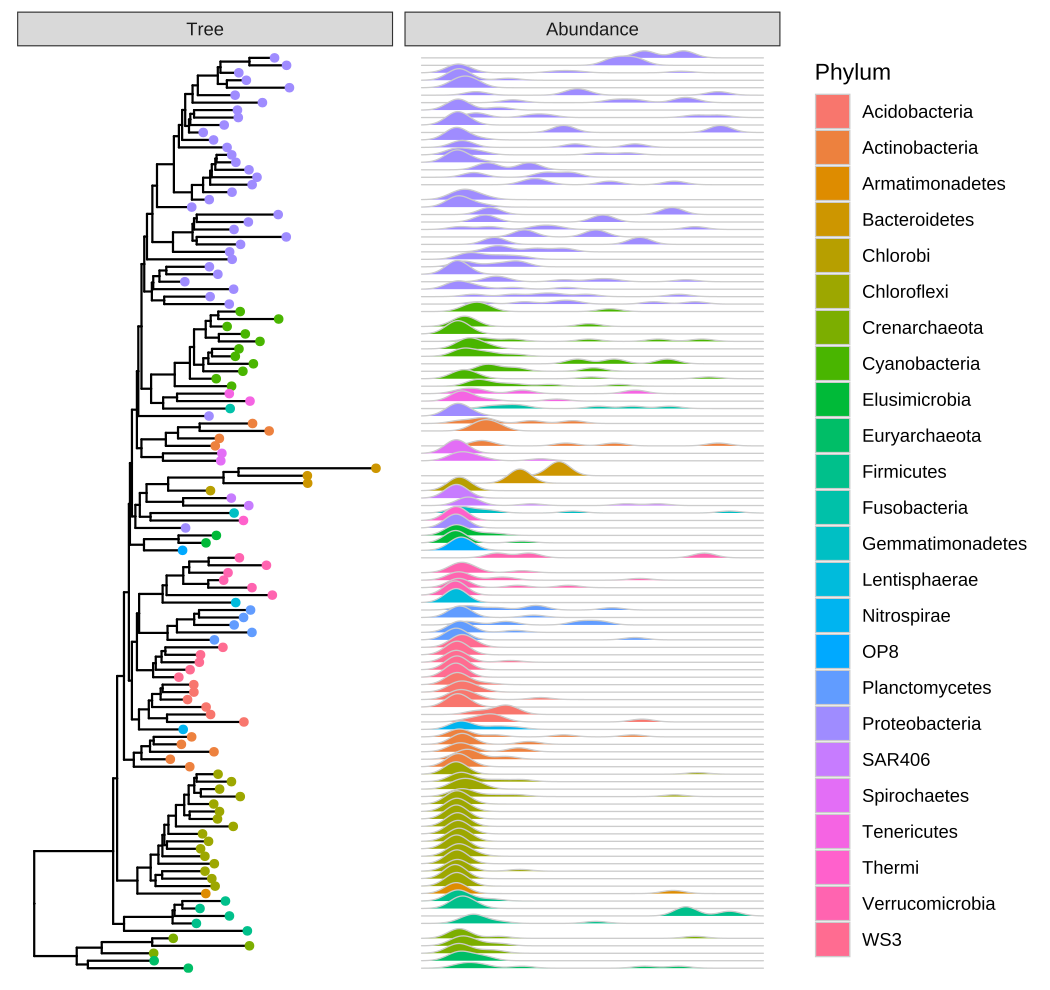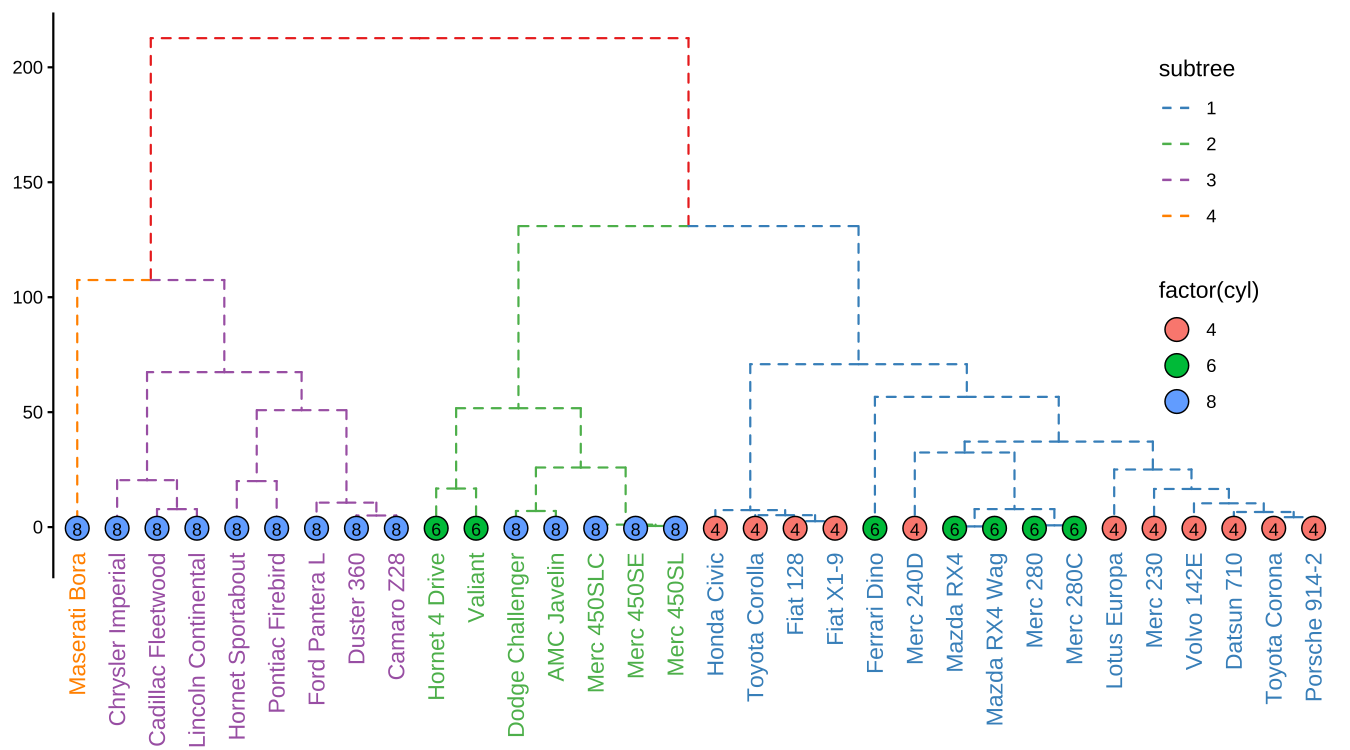# 9 ggtree for Other Tree-like Objects

## 9.1 ggtree for Phylogenetic Tree Objects

The treeio packages allow parsing evolutionary inferences from several software outputs and linking external data to the tree structure. It serves as an infrastructure to bring evolutionary data to the R community. The ggtree package works seamlessly with treeio to visualize tree-associated data to annotate the tree. The ggtree package is a general tool for tree visualization and annotation and it fits the ecosystem of R packages. Most of the S3/S4 tree objects defined by other R packages are also supported by ggtree, including phylo (session 4.2), multiPhylo (session 4.4), phylo4, phylo4d, phyloseq, and obkData. With ggtree, we are able to generate more complex tree graphs which is not possible or easy to do with other packages. For example, the visualization of the phyloseq object in Figure 9.4 is not supported by the phyloseq package. The ggtree package also extends the possibility of linking external data to these tree objects .

### 9.1.1 The phylo4 and phylo4d objects

The phylo4 and phylo4d are defined in the phylobase package. The phylo4 object is an S4 version of phylo, while phylo4d extends phylo4 with a data frame that contains trait data. The phylobase package provides a plot() method, which is internally called the treePlot() function, to display the tree with the data. However, there are some restrictions of the plot() method, it can only plot numeric values for tree-associated data as bubbles and cannot generate figure legend. Phylobase doesn’t implement a visualization method to display categorical values. Using associated data as visual characteristics such as color, size, and shape, is also not supported. Although it is possible to color the tree using associated data, it requires users to extract the data and map them to the color vector manually followed by passing the color vector to the plot method. This is tedious and error-prone since the order of the color vector needs to be consistent with the edge list stored in the object.

The ggtree package supports phylo4d object and all the associated data stored in the phylo4d object can be used directly to annotate the tree (Figure 9.1).

library(phylobase)
data(geospiza_raw)
g1 <- as(geospiza_raw$tree, "phylo4") g2 <- phylo4d(g1, geospiza_raw$data, missing.data="warn")

d1 <- data.frame(x = seq(1.1, 2, length.out = 5),
lab = names(geospiza_raw$data)) p1 <- ggtree(g2) + geom_tippoint(aes(size = wingL), x = d1$x, shape = 1) +
geom_tippoint(aes(size = tarsusL), x = d1$x, shape = 1) + geom_tippoint(aes(size = culmenL), x = d1$x, shape = 1) +
geom_tippoint(aes(size = beakD),   x = d1$x, shape = 1) + geom_tippoint(aes(size = gonysW), x = d1$x, shape = 1) +
scale_size_continuous(range = c(3,12), name="") +
geom_text(aes(x = x, y = 0, label = lab), data = d1, angle = 45) +
geom_tiplab(offset = 1.3) + xlim(0, 3) +
theme(legend.position = c(.1, .75)) + vexpand(.05, -1)

## users can use as.treedata(g2) to convert g2 to a treedata object
## and use get_tree_data() function to extract the associated data

p2 <- gheatmap(ggtree(g1), data=geospiza_raw$data, colnames_angle=45) + geom_tiplab(offset=1) + hexpand(.2) + vexpand(.05, -1) + theme(legend.position = c(.1, .75)) aplot::plot_list(p1, p2, ncol=2, tag_levels='A')FIGURE 9.1: Visualizing phylo4d data using ggtree. Reproduce the output of the plot() method provided in the phylobase package (A). Visualize the trait data as a heatmap which is not supported in the phylobase package (B). ### 9.1.2 The phylog object The phylog is defined in the ade4 package. The package is designed for analyzing ecological data and provides newick2phylog(), hclust2phylog(), and taxo2phylog() functions to create phylogeny from Newick string, hierarchical clustering result, or a taxonomy (see also the MicrobiotaProcess package described in Chapter 11). The phylog object is also supported by ggtree as demonstrated in Figure 9.2. library(ade4) data(taxo.eg) tax <- as.taxo(taxo.eg[]) names(tax) <- c("genus", "family", "order") print(tax) ## genus family order ## esp3 g1 fam1 ORD1 ## esp1 g1 fam1 ORD1 ## esp2 g1 fam1 ORD1 ## esp4 g1 fam1 ORD1 ## esp5 g1 fam1 ORD1 ## esp6 g1 fam1 ORD1 ## esp7 g1 fam1 ORD1 ## esp8 g2 fam2 ORD2 ## esp9 g3 fam2 ORD2 ## esp10 g4 fam3 ORD2 ## esp11 g5 fam3 ORD2 ## esp12 g6 fam4 ORD2 ## esp13 g7 fam4 ORD2 ## esp14 g8 fam5 ORD2 ## esp15 g8 fam5 ORD2 tax.phy <- taxo2phylog(as.taxo(taxo.eg[])) print(tax.phy) ## Phylogenetic tree with 15 leaves and 16 nodes ##$class: phylog
## $call: taxo2phylog(taxo = as.taxo(taxo.eg[])) ##$tre: ((((esp3,esp1,esp2,esp4,e...15)l1g8)l2fam5)l3ORD2)Root;
##
##         class   length
## $leaves numeric 15 ##$nodes  numeric 16
## $parts list 16 ##$paths  list    31
## $droot numeric 31 ## content ##$leaves length of the first preceeding adjacent edge
## $nodes length of the first preceeding adjacent edge ##$parts  subsets of descendant nodes
## $paths path from root to node or leave ##$droot  distance to root
ggtree(tax.phy) + geom_tiplab() +
geom_nodelab(geom='label') + hexpand(.05)FIGURE 9.2: Visualizing a phylog tree object.

### 9.1.3 The phyloseq object

The phyloseq class defined in the phyloseq package was designed for storing microbiome data, including a phylogenetic tree, associated sample data, and taxonomy assignment. It can import data from popular pipelines, such as QIIME , mothur , dada2 and PyroTagger , etc. The ggtree supports visualizing the phylogenetic tree stored in the phyloseq object and related data can be used to annotate the tree as demonstrated in Figures 9.3 and 9.4.

library(phyloseq)
library(scales)

data(GlobalPatterns)
GP <- prune_taxa(taxa_sums(GlobalPatterns) > 0, GlobalPatterns)
GP.chl <- subset_taxa(GP, Phylum=="Chlamydiae")

ggtree(GP.chl) +
geom_nodelab(aes(label=label), hjust=-.05, size=3.5) +

geom_point(aes(x=x+hjust, color=SampleType, shape=Family,
size=Abundance), na.rm=TRUE) +
geom_tiplab(aes(label=Genus), hjust=-.35) +
scale_size_continuous(trans=log_trans(5)) +
theme(legend.position="right") + hexpand(.4)FIGURE 9.3: Visualizing a phyloseq tree object. This example mimics the output of the plot_tree() function provided in the phyloseq package.

Figure 9.3 reproduces the output of the phyloseq::plot_tree() function. Users of phyloseq will find ggtree useful for visualizing microbiome data and for further annotation since ggtree supports high-level annotation using the grammar of graphics and can add tree data layers that are not available in phyloseq.

library(ggridges)

data("GlobalPatterns")
GP <- GlobalPatterns
GP <- prune_taxa(taxa_sums(GP) > 600, GP)
sample_data(GP)\$human <- get_variable(GP, "SampleType") %in%
c("Feces", "Skin")

mergedGP <- merge_samples(GP, "SampleType")
mergedGP <- rarefy_even_depth(mergedGP,rngseed=394582)
mergedGP <- tax_glom(mergedGP,"Order")

melt_simple <- psmelt(mergedGP) %>%
filter(Abundance < 120) %>%
select(OTU, val=Abundance)

ggtree(mergedGP) +
geom_tippoint(aes(color=Phylum), size=1.5) +
geom_facet(mapping = aes(x=val,group=label,
fill=Phylum),
data = melt_simple,
geom = geom_density_ridges,
panel="Abundance",
color='grey80', lwd=.3) +
guides(color = guide_legend(ncol=1))FIGURE 9.4: Phylogenetic tree with OTU abundance densities. Tips were colored by Phylum, and the corresponding abundances across different samples were visualized as density ridgelines and sorted according to the tree structure.

This example uses microbiome data provided in the phyloseq package and density ridgeline is employed to visualize species abundance data. The geom_facet() layer automatically re-arranges the abundance data according to the tree structure, visualizes the data using the specified geom function, i.e., geom_density_ridges(), and aligns the density curves with the tree as demonstrated in Figure 9.4. Note that data stored in the phyloseq object is visible to ggtree() and can be used directly in tree visualization (Phylum was used to color tips and density ridgelines in this example). The source code of this example was firstly published in the supplemental file of .

## 9.2 ggtree for Dendrograms

A dendrogram is a tree diagram to display hierarchical clustering and classification/regression trees. In R, we can calculate a hierarchical clustering using the function hclust().

hc <- hclust(dist(mtcars))
hc
##
## Call:
## hclust(d = dist(mtcars))
##
## Cluster method   : complete
## Distance         : euclidean
## Number of objects: 32

The hclust object describes the tree produced by the clustering process. It can be converted to dendrogram object, which stores the tree as deeply-nested lists.

den <- as.dendrogram(hc)
den
## 'dendrogram' with 2 branches and 32 members total, at height 425.3

The ggtree package supports most of the hierarchical clustering objects defined in the R community, including hclust and dendrogram as well as agnes, diana, and twins that are defined in the cluster package, and the pvclust object defined in the pvclust package (Table C.2). Users can use ggtree(object) to display its tree structure, and use other layers and utilities to customize the graph and of course, add annotations to the tree.

The ggtree provides layout_dendrogram() to layout the tree top-down, and theme_dendrogram() to display tree height (similar to theme_tree2() for phylogenetic tree) as demonstrated in Figure 9.5 (see also the example in (Yu, 2020)).

clus <- cutree(hc, 4)
g <- split(names(clus), clus)

p <- ggtree(hc, linetype='dashed')
clades <- sapply(g, function(n) MRCA(p, n))

d <- data.frame(label = names(clus),
cyl = mtcars[names(clus), "cyl"])

p %<+% d +
layout_dendrogram() +
geom_tippoint(aes(fill=factor(cyl), x=x+.5),
size=5, shape=21, color='black') +
geom_tiplab(aes(label=cyl), size=3, hjust=.5, color='black') +
geom_tiplab(angle=90, hjust=1, offset=-10, show.legend=FALSE) +
scale_color_brewer(palette='Set1', breaks=1:4) +
theme_dendrogram(plot.margin=margin(6,6,80,6)) +
theme(legend.position=c(.9, .6))FIGURE 9.5: Visualizing dendrogram. Use cutree() to split the tree into several groups and groupClade() to assign this grouping information. The tree was displayed in the classic top-down layout with branches colored by the grouping information and the tips were colored and labeled by the number of cylinders.

## 9.3 ggtree for Tree Graph

The tree graph (as an igraph object) can be converted to a phylo object using as.phylo() method provided in the treeio package (Table C.2). The ggtree supports directly visualizing tree graph as demonstrated in Figure 9.6. Note that currently not all igraph objects can be supported by ggtree. Currently, it can only be supported when it is a tree graph.

library(igraph)
g <- graph.tree(40, 3)
arrow_size <- unit(rep(c(0, 3), times = c(27, 13)), "mm")
ggtree(g, layout='slanted', arrow = arrow(length=arrow_size)) +
geom_point(size=5, color='steelblue', alpha=.6) +
geom_tiplab(hjust=.5,vjust=2) + layout_dendrogram()FIGURE 9.6: Visualizing a tree graph. The lines with arrows indicate the relationship between the parent node and the child node. All nodes were indicated by steelblue circle points.

## 9.4 ggtree for Other Tree-like Structures

The ggtree package can be used to visualize any data in a hierarchical structure. Here, we use the GNI (Gross National Income) numbers in 2014 as an example. After preparing an edge list, that is a matrix or data frame that contains two columns indicating the relationship of parent and child nodes, we can use the as.phylo() method provided by the treeio package to convert the edge list to a phylo object. Then it can be visualized using ggtree with associated data. In this example, the population was used to scale the size of circle points for each country (Figure 9.7).

library(treeio)
library(ggplot2)
library(ggtree)

data("GNI2014", package="treemap")
n <- GNI2014[, c(3,1)]
n[,1] <- as.character(n[,1])
n[,1] <- gsub("\\s\$$.*\$$", "", n[,1])

w <- cbind("World", as.character(unique(n[,1])))

colnames(w) <- colnames(n)
edgelist <- rbind(n, w)

y <- as.phylo(edgelist)
ggtree(y, layout='circular') %<+% GNI2014 +
aes(color=continent) + geom_tippoint(aes(size=population), alpha=.6) +
geom_tiplab(aes(label=country), offset=.05, size=3) +
xlim(NA, 3)FIGURE 9.7: Visualizing data in any hierarchical structure. Hierarchical data represented as nodes connected by edges can be converted to a phylo object and visualized by ggtree to explore their relationships or other properties that are associated with the relationships.

## 9.5 Summary

The ggtree supports various tree objects defined in the R language and extension packages, which makes it very easy to integrate ggtree into existing pipelines. Moreover, ggtree allows external data integration and exploration of these data on the tree, which will greatly promote the data visualization and result in interpretation in the downstream analysis of existing pipelines. Most importantly, the support for converting edge list to a tree object enables more tree-like structures to be incorporated into the framework of treeio and ggtree. This will enable more tree-like structures and related heterogeneous data in different disciplines to be integrated and visualized through treeio and ggtree, which facilitates integrated analysis and comparative analysis to discover more systematic patterns and insights.